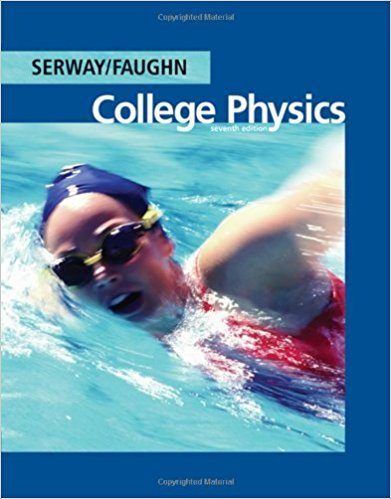×
Get Full Access to College Physics - 7 Edition - Chapter 4 - Problem 4.17
Get Full Access to College Physics - 7 Edition - Chapter 4 - Problem 4.17

×

# A 150-N bird feeder is supported by three cables as shownISBN: 9780495113690 154

## Solution for problem 4.17 Chapter 4

College Physics | 7th Edition

• Textbook Solutions
• 2901 Step-by-step solutions solved by professors and subject experts
• Get 24/7 help from StudySoup virtual teaching assistantsCollege Physics | 7th Edition

4 5 1 291 Reviews
16
5
Problem 4.17

A 150-N bird feeder is supported by three cables as shown in Figure P4.17. Find the tension in each cable.

Step-by-Step Solution:
Step 1 of 3

Lectures 1 2 and 3 Average Speed & Average Velocity vavgdistancetime=dt *speed is a scalar (magnitude with no direction vavgdisplacementtime= rt * velocity is a vector (has direction) → UNITS: ms vavg and r point in direction of motion vx= xf-x0t → xf=x0+t(vx) *with constant velocity  Negative slope = negative velocity  Steeper slope = faster speed Acceleration aavgvt *rate of change of velocity (slowing, speeding, changing direction) aavgv - n+1 n → UNITS: ms 2 → If v and a are point in the same direction, the object is speeding up afree fall0 ms2 → 1 dimensional acceleration down a slope: as= g(sin) Ki

Step 2 of 3

Step 3 of 3

##### ISBN: 9780495113690

Unlock Textbook Solution